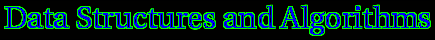"Computer science is no more about computers than astronomy is about telescopes."

E. W. DijkstraSpecific Course Material for COMP-251

as taught by Godfried ToussaintChapter Index:### 2.  The Correctness of Algorithms (Proof Techniques):

1. Notes on methods of proof
2. Notes on how to do proofs
3. More on proof methods
4. Classic fallacies
5. Constructive Proofs:
7. Induction Proofs:
8. Induction Algorithm Design:
1. Polynomial evaluation
9. Many nice examples of different types of proofs
10. On Proofs in Mathematics
12. Writing Down Proofs
13. The Nuts and Bolts of Proofs
14. Logical systems
15. Common errors in mathematics
16. Proofs and proof strategies
3.  Linear Data Structures: 4. Graphs:
1. Interactive Introduction to Graph Theory
2. Introduction to Graphs (Luc Devroye's Notes)
3. Graph isomorphism
4. Graph planarity
5. Graph data structures:
6. Graph Algorithm Animations
7. Graph Theory Web Tutorials
8. Graph Glossary
9. Proximity graphs:
10. Hamiltonian Tours:
1. Hamiltonian paths in tournaments
2. Hamiltonian cycles in dense graphs:
1. Ore's Theorem
1. Backward induction proof
3. Dirac's Theorem
4. The Hamiltonian Page
5. Great tutorial on Hamilton circuits
6. Sufficient conditions for Hamiltonian cycles
7. Hamiltonian circuits of point sets with specific properties (polygonizations)
11. Minimum Spanning Trees:
12. 19 Proofs of Euler's Theorem
13. Graph and Map Coloring:
14. The Konigsberg Bridge Problem
15. Planarity and the Torus
16. The rotating-caliper graph
17. The Travelling Salesman Problem:
18. Graph drawing
5. Trees:
1. Introduction to Trees:
2. Tree Algorithm Animations
3. Binary trees.
5. Balanced trees:
1. Tutorial on 2-4 trees
2. 2-4 Trees (also AVL and Red-Black trees)
6. Heaps:
7. Huffman trees and data compression:
1. Data compression
2. Text compression (Steve Skiena)
3. Morse Code
4. Introduction to probability and information theory
5. Entropy
6. Markov models of natural language (monkeys and typewriters)
7. Spelling correction programs.
8. Decision trees
9. Game trees:
1. Play Checkers with Chinook (The World Champion)
2. Play Othello with Keyano and other programs
3. Play Chess with The Turk
4. Game trees
5. Other Games, Toys and Puzzles
6. Clever games for clever people
6. Searching Algorithms:
1. Sequential search
2. Binary search:
1. Creating Binary Search Trees (Java applet)
2. Searching in a Binary Search Tree (Java applet demo)
3. The Number-Guessing Game
3. Maze searching:
4. Breadth-first search (Luc Devroye's class notes)
5. Interpolation search
6. Orthogonal range searching
7. Nearest Neighbor Search:
1. Projection methods
2. Access Trees
3. Voronoi diagram methods
8. Closest-pair searching in the plane
9. Branch-and-bound
10. The A* algorithm
7.  Plane-Sweep Algorithms:
1. Closest pair problem
2. Line segment intersections
8. Greedy Algorithms, Hill-Climbing, and Diameter Algorithms:
1. Greedy algorithms
2. The Rotating Calipers
1. The Rotating Caliper Page of Hormoz Pirzadeh (with an awsome Java applet!)
2. The Reuleaux triangle (Eric's Treasure Trove)
3. The Reuleaux triangle (The Geometry Junkyard
3. Complexity, convexity and unimodality
4. Hill climbing algorithms
5. Quick sort
6. Quick hull
9.  Divide & Conquer Algorithms:
1. Sorting
2. Finding closest pair
3. Convex hulls
10. On-Line Algorithms:
1. Convex hulls of polygons
2. Convex hulls of points
11.  Real-Time Algorithms:
1. Convex hulls of polygons
2. Convex hulls of points
12. Elimination Methods:
1. Convex hulls of point sets
13.  Distributive Methods:
1. Distributive sorting
2. Bucketing methods
1. Bucket sort
14.  Prune & Search Methods: 15. Linear Programming:
1. Introduction to Linear Programming and the Simplex Algorithm
2. Megiddo's linear time algorithm
3. Linear programming and optimization problems (with Java applet)
16.  Probabilistic Algorithms:
1. Randomization
2. Monte-Carlo Algorithms
3. Las-Vegas Algorithms
4. Randomized convex hull
5. Probability and Random Number Generation
6. Monte Carlo Simulation:
17.  Approximation Algorithms: 18.  Parallel Algorithms:
1. Parallel Algorithm Animations
2. Real and Artificial Neurons
3. Threshold logic units.
4. Dr. Gurney's course on neural networks.
5. Perceptrons & neural networks (learning machines).
6. Sharpening, the Laplacian and lateral inhibition in neural networks (PDF)

## 19.1 Numbers

19.2 Sorting Algorithms
1. Sorting Numbers:
1. Sorting Algorithm Animations
2. Selection sort tutorial
3. Bubble sort tutorial
4. Heap sort tutorial
5. Merge sort tutorial (Divide and Conquer)
6. Bucket-Sorting and Floor Functions (PostScript file), (PDF file)
7. Quicksort with nice applet! (in place version and comparison to heapsort)
8. Expected analysis of randomized quicksort
9. Random binary search trees and Quicksort
2. Selection and order statistics
3. More Sorting Algorithm Animations (Applets also sort your own set of numbers!)
4. Sorting Code
20. Geometric Algorithms:
1. Polygon Triangulation:
1. History of Triangulation Algorithms
2. Ears and Mouths of Polygons:
1. Ian Garton's Tutorial on cutting ears and stuffing mouths (with interactive Java applets)
2. Simple polygons have ears and mouths
3. Meisters' Two-Ears Theorem
4. The one-mouth theorem:
3. Diagonal insertion
4. Prune-and-Search:
5. The Graham Scan triangulates simple polygons (PostScript)
6. Fast Polygon Triangulation in Practice:
1. Efficient polygon triangulation algorithms. Includes counter-examples to many published algorithms. (PostScript)
2. The Art-Gallery Theorem (with interactive applet)
3. The Minimal Spanning Tree applet
4. Geometric Algorithm Animations
5. Polygons: crossing, simple and convex.
6. Polygonizing Sets of Points:
7. The area of a polygon.
8. Determining if a point is inside a polygon.
9. Smoothing polygons and polygonal waveforms.
10. Convex Hulls:
11. Voronoi Diagrams:
21.  Sequence Comparison: 22.  Computational Aspects of Musical Rhythm: 21.  Arithmetic Operations: